# 3. Let I be a left ideal of R and let (: R) reRRCI (a) : R) is an ideal of R. If l is regular, th...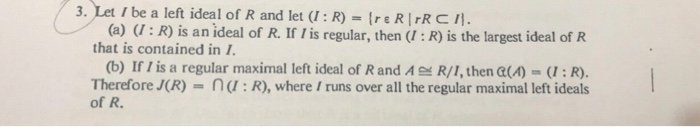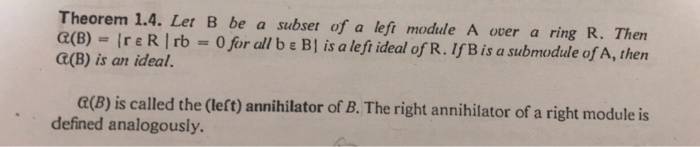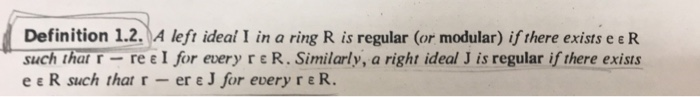3. Let I be a left ideal of R and let (: R) reRRCI (a) : R) is an ideal of R. If l is regular, then (: R) is the largest ideal of R that is contained in 1 (b) If I is a regular maximal left ideal of Rand AR/I, then (A(R). Therefore J(R) na:R), where /runs over all the regular maximal left ideals of R.
Theorem 1.4. Let B be a subset of a left module A over a ring R. Thern a(B)-Ire R | rb-0 for all b ε BI is a left ideal of R. If B is a submodule of A, then C(B) is an ideal. e(B) is called the (left) annihilator of B. The right annihilator of a right module is defined analogously.
Definition 1.2.A left ideal I in a ring R is regular (or modular) if there exists e e R suchthat-r-re ε| for every r ε R. Similarly, a right ideal J is regular if there exists e e . R such that r-ere J for every re R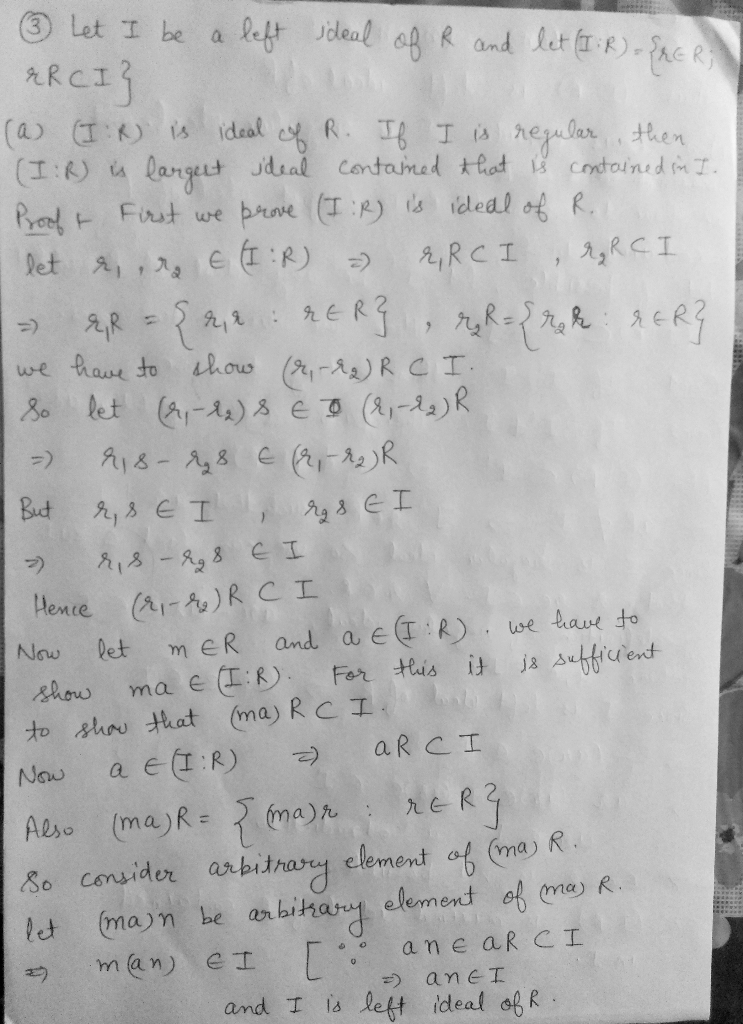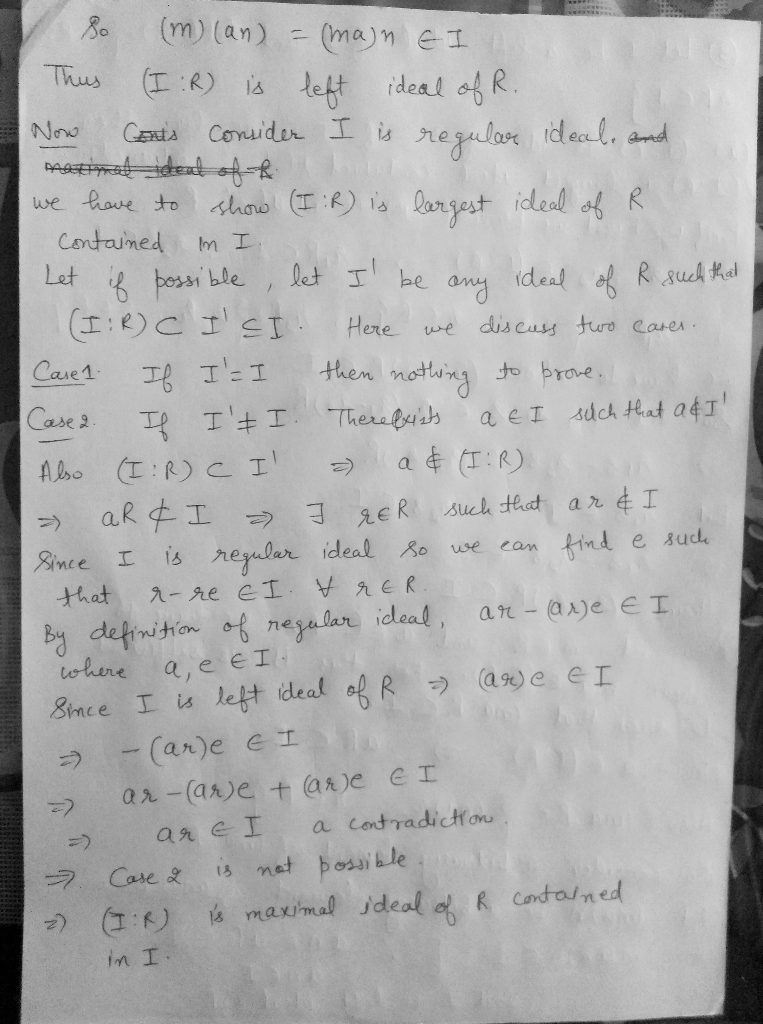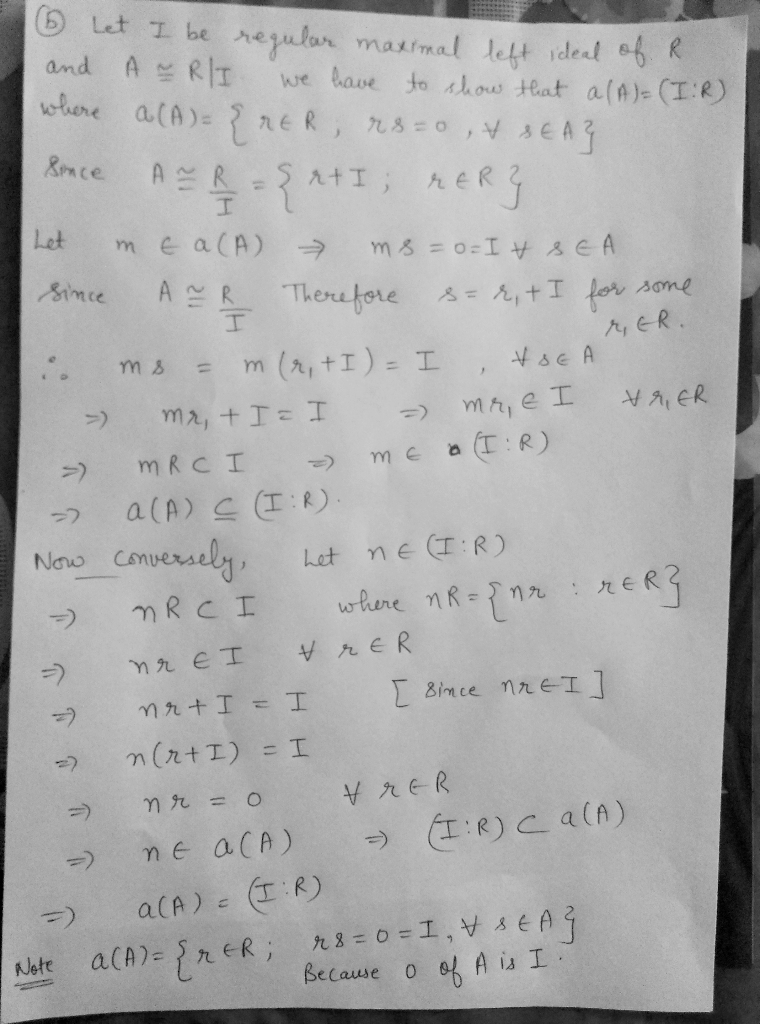##### Add Answer of: 3. Let I be a left ideal of R and let (: R) reRRCI (a) : R) is an ideal of R. If l is regular, th...
Similar Homework Help Questions
• ### thanks Let I be a proper ideal of a commutative ring R with 1. Prove that...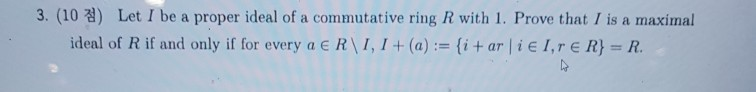thanks Let I be a proper ideal of a commutative ring R with 1. Prove that I is a maximal 3. (10 ideal of R if and only if for every a e R\I, I+(a) : {i+ ar i e I,rE R} = R. Let I be a proper ideal of a commutative ring R with 1. Prove that I is a maximal 3. (10 ideal of R if and only if for every a e R\I, I+(a) : {i+...

• ### 66. Let R be a commutative ring with identity. An ideal I of R is irreducible if it cannot be expressed as the i...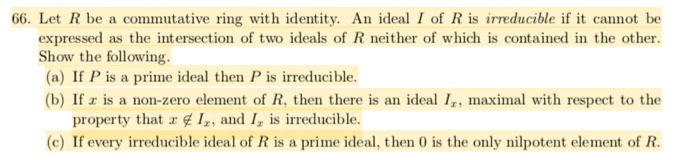66. Let R be a commutative ring with identity. An ideal I of R is irreducible if it cannot be expressed as the intersection of two ideals of R neither of which is contained in the other. the following. (a) If P is a prime ideal then P is irreducible. (b) If z is a non-zero element of R, then there is an ideal I, maximal with respect to the property that r gI, and I is irreducible. (c) If...

• ### 74. Let R be a commutative ring with identity such that not every ideal is a principal ideal principal ideal. (b)...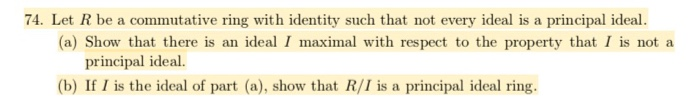74. Let R be a commutative ring with identity such that not every ideal is a principal ideal principal ideal. (b) If I is the ideal of part (a), show that R/I is a principal ideal ring 74. Let R be a commutative ring with identity such that not every ideal is a principal ideal principal ideal. (b) If I is the ideal of part (a), show that R/I is a principal ideal ring

• ### Please answer all parts. Thank you! 20. Let R be a commutative ring with identity. We define a multiplicative subset of R to be a subset S such that 1 S and ab S if a, b E S. Define a relation ~ on R...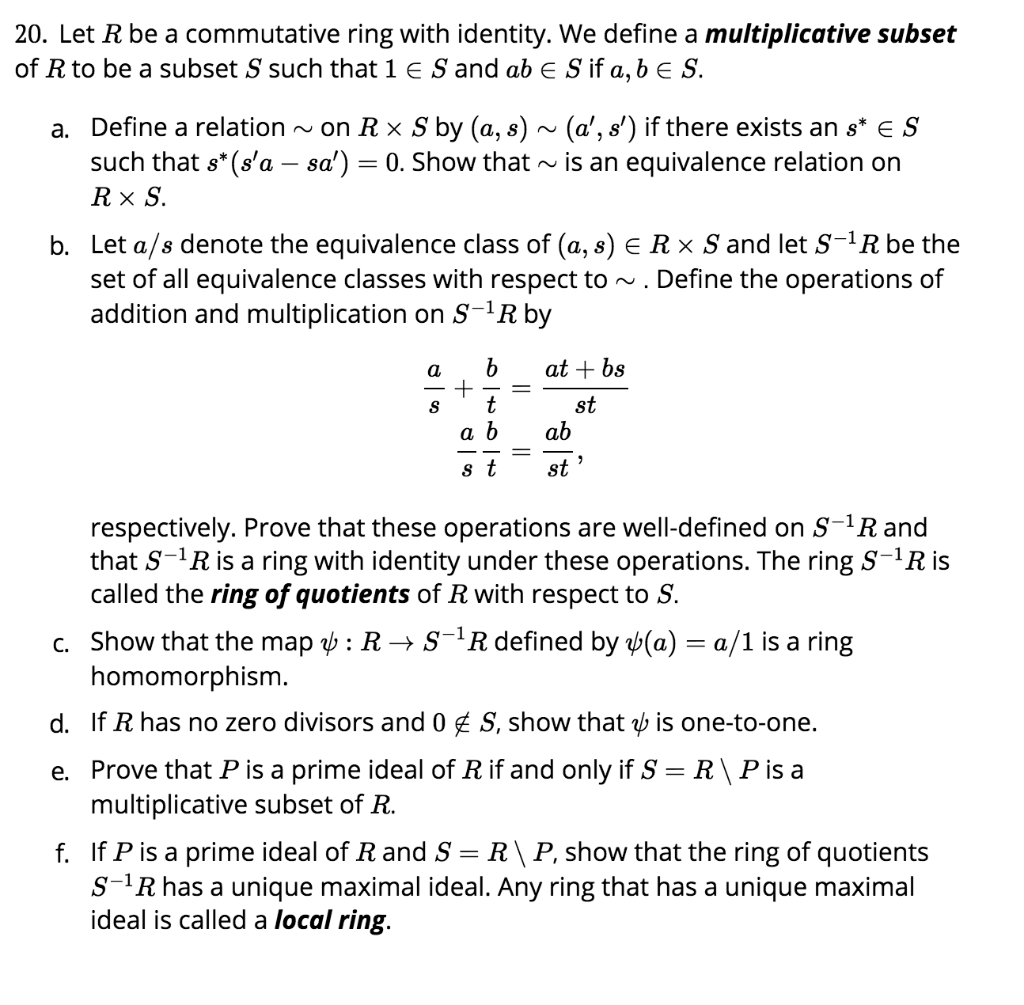Please answer all parts. Thank you! 20. Let R be a commutative ring with identity. We define a multiplicative subset of R to be a subset S such that 1 S and ab S if a, b E S. Define a relation ~ on R × S by (a, s) ~ (a, s') if there exists an s"e S such that s* (s,a-sa,) a. 0. Show that ~ is an equivalence relation on b. Let a/s denote the equivalence class...

• ### Can you please provide clear and step by step solution for both 3 and 4. Thanks...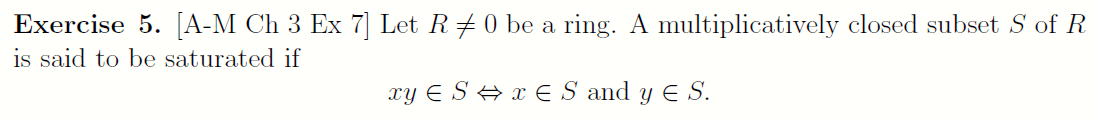Can you please provide clear and step by step solution for both 3 and 4. Thanks :) Exercise 5. [A-M Ch 3 Ex 7] Let R#0 be a ring. A multiplicatively closed subset S of R is said to be saturated if XY ES #xe S and y E S. 1. Let I be the collection of all multiplicatively closed subsets of R such that 0 € S. Show that I has maximal elements, and that Se & is maximal...

• ### Solve problem 2 using the priblem 1 . Question is taken from Ring theory dealing with ideals and ...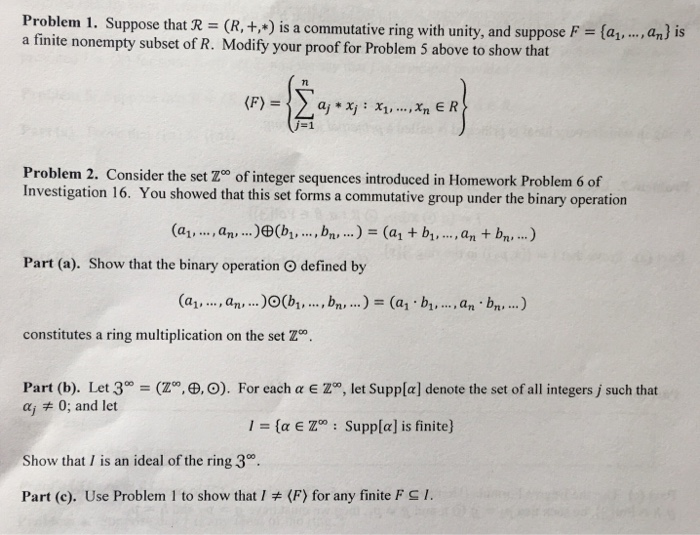Solve problem 2 using the priblem 1 . Question is taken from Ring theory dealing with ideals and generating sets for ideals. Problem 1. Suppose that R (R,+ Jis a commutative ring with unity, and suppose F- (a,,. , a } is a finite nonempty subset of R. Modify your proof for Problem 5 above to show that 7n j-1 Problem 2. Consider the set Zo of integer sequences introduced in Homework Problem 6 of Investigation 16. You showed that...

• ### THEOREM 3.1 Let r be a regular expression. Then there exists some nondeteministic finite accepter that...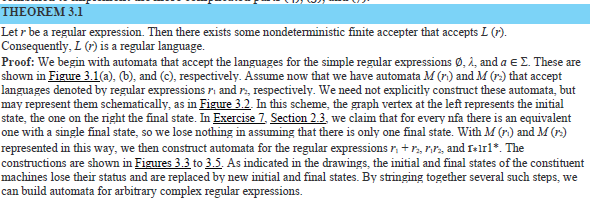THEOREM 3.1 Let r be a regular expression. Then there exists some nondeteministic finite accepter that accepts L (r) Consequently, L () is a regular language. Proof: We begin with automata that accept the languages for the simple regular expressions ø, 2, and a E . These are shown in Figure 3.1(a), (b), and (c), respectively. Assume now that we have automata M (r) and M (r) that accept languages denoted by regular expressions ri and r respectively. We need...

• ### ring over Q in countably many variables. Let I be the ideal of R generated by all polynomials -Pi where p; is the ith prime. Let RnQ1,2, 3, n] be the polyno- mial ring over Q in n variables. Let...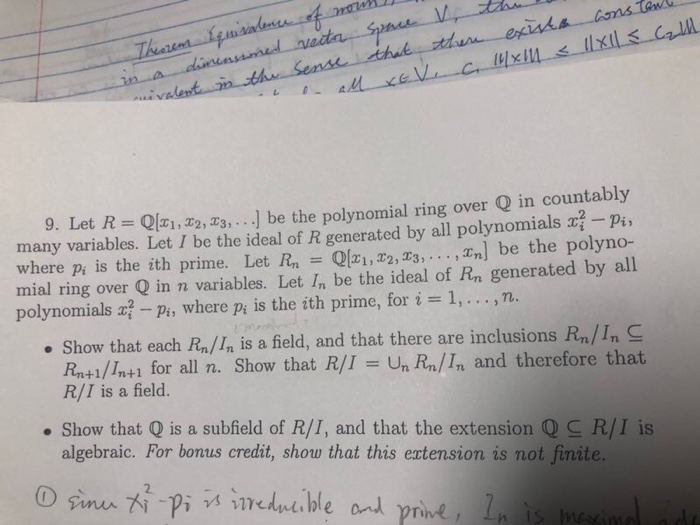ring over Q in countably many variables. Let I be the ideal of R generated by all polynomials -Pi where p; is the ith prime. Let RnQ1,2, 3, n] be the polyno- mial ring over Q in n variables. Let In be the ideal of Rn generated by all ] be the polynomial rin 9. Let R = QlX1,22.Zg, 2 polynomials -pi, where pi is the ith prime, for i1,.,n. . Show that each Rn/In is a field, and that...

• ### (a) Let R be a commutative ring. Given a finite subset {ai, a2, , an} of R, con- sider the set {rial + r202 + . . . + rnan I ri, r2, . . . , rn є R), which we denote by 〈a1, a2 , . . . , Prove that...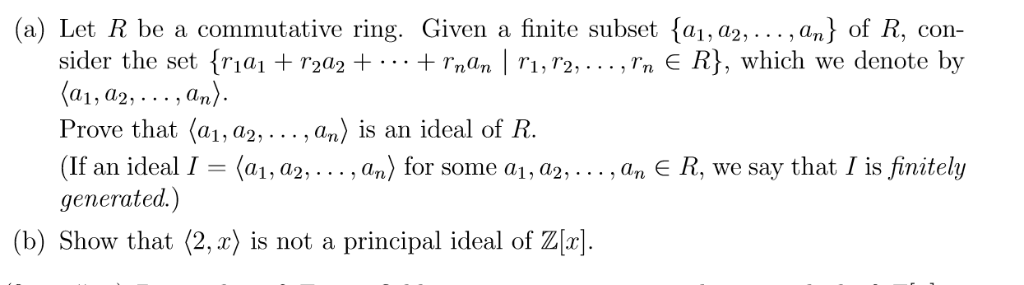(a) Let R be a commutative ring. Given a finite subset {ai, a2, , an} of R, con- sider the set {rial + r202 + . . . + rnan I ri, r2, . . . , rn є R), which we denote by 〈a1, a2 , . . . , Prove that 〈a1, a2, . . . , an〉 įs an ideal of R. (If an ideal 1 = 〈a1, аг, . . . , an) for some a,...

• ### Topology (b) Let S denote the subset of co consisting of sequences with rational entries of which at most finitely many are nonzero. (i) Show that S is dense in co with the sup norm. [Hint: Show that...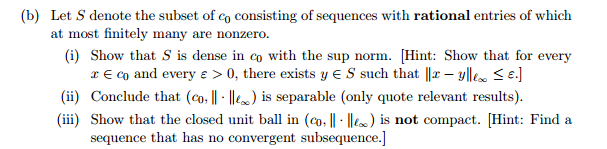Topology (b) Let S denote the subset of co consisting of sequences with rational entries of which at most finitely many are nonzero. (i) Show that S is dense in co with the sup norm. [Hint: Show that for every r E co and every ε > 0, there exists y S such that llx-yI100 < ε.j (ii) Conclude that (co, ll . 114) is separable (only quote relevant results) (iii) Show that the closed unit ball in (a-II ·...

Need Online Homework Help?